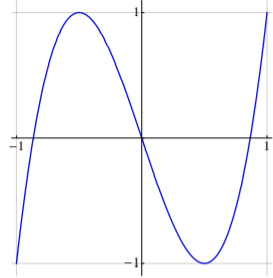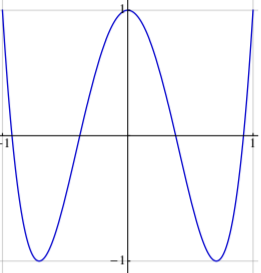# nth degree polynomials inside square.$y=4x^3-3x$$y=8x^4 - 8x^2 +1$

The two pictures are examples of third and fourth degree polynomials 'just fitting' inside a square of side length 2 and centered about the origin. These surprisingly have integer coefficients.

What can be said about polynomials of a higher degree?

Here are degrees 2-5:

$2x^2-1$

$4x^3-3x$

$8x^4-8x^2+1$

$16x^5-20x^3+5x$ (By trial and error)

It is easy to show that the coefficients must sum to 1 and it appears the leading coefficient increases by powers of 2Note by Chris Sapiano
7 months, 1 week ago

This discussion board is a place to discuss our Daily Challenges and the math and science related to those challenges. Explanations are more than just a solution — they should explain the steps and thinking strategies that you used to obtain the solution. Comments should further the discussion of math and science.

When posting on Brilliant:

• Use the emojis to react to an explanation, whether you're congratulating a job well done , or just really confused .
• Ask specific questions about the challenge or the steps in somebody's explanation. Well-posed questions can add a lot to the discussion, but posting "I don't understand!" doesn't help anyone.
• Try to contribute something new to the discussion, whether it is an extension, generalization or other idea related to the challenge.
• Stay on topic — we're all here to learn more about math and science, not to hear about your favorite get-rich-quick scheme or current world events.

MarkdownAppears as
*italics* or _italics_ italics
**bold** or __bold__ bold
- bulleted- list
• bulleted
• list
1. numbered2. list
1. numbered
2. list
Note: you must add a full line of space before and after lists for them to show up correctly
paragraph 1paragraph 2

paragraph 1

paragraph 2

[example link](https://brilliant.org)example link
> This is a quote
This is a quote
    # I indented these lines
# 4 spaces, and now they show
# up as a code block.

print "hello world"
# I indented these lines
# 4 spaces, and now they show
# up as a code block.

print "hello world"
MathAppears as
Remember to wrap math in $$ ... $$ or $ ... $ to ensure proper formatting.
2 \times 3 $2 \times 3$
2^{34} $2^{34}$
a_{i-1} $a_{i-1}$
\frac{2}{3} $\frac{2}{3}$
\sqrt{2} $\sqrt{2}$
\sum_{i=1}^3 $\sum_{i=1}^3$
\sin \theta $\sin \theta$
\boxed{123} $\boxed{123}$

## Comments

Sort by:

Top Newest
- 7 months, 1 week ago

Log in to reply

Interesting that there is this connection, why is this so?

- 7 months, 1 week ago

Log in to reply

I guess it's just a corollary of Chebyshev polynomials. I can't verbally explain this.

- 7 months, 1 week ago

Log in to reply

×

Problem Loading...

Note Loading...

Set Loading...